iPhone Screenshots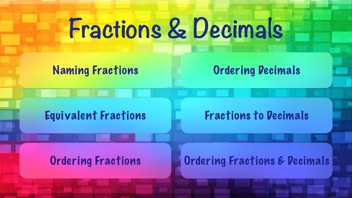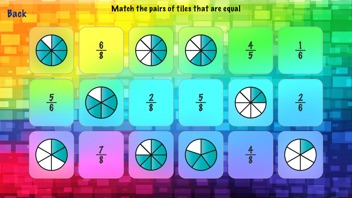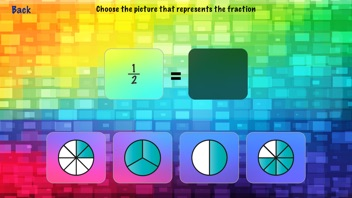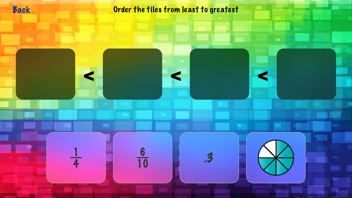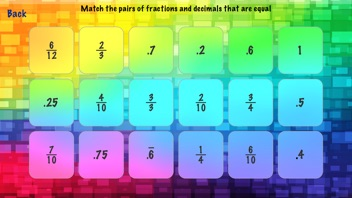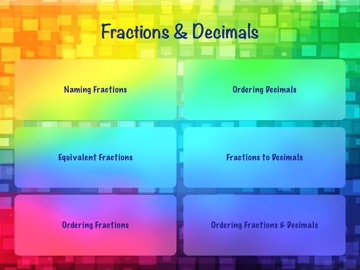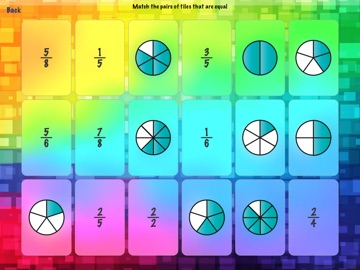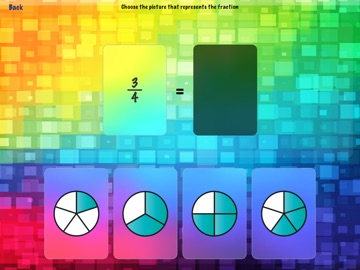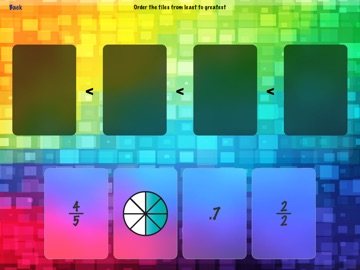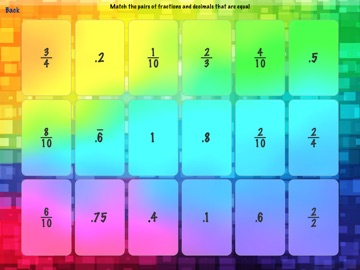Description

Fractions & Decimals has fun and simple activities to practice a range of fraction and decimal concepts such as: naming fractions, equivalent fractions, ordering fractions and decimals, and equivalent fractions and decimals.
The app has the following activities:
Naming Fractions:
Multiple Choice Mode: Choose the picture that represents the fraction
Matching Mode: Match fractions to the pictures of the fractions
Equivalent Fractions:
Multiple Choice Mode: Choose the fraction equivalent to the given fraction
Matching Mode: Match the pairs of equivalent fractions
Ordering Fractions:
Order fractions from least to greatest (2 levels)
Ordering Decimals:
Order decimals from least to greatest
Fractions to Decimals:
Multiple Choice Mode: Choose the decimal that is equal to the given fraction (2 levels)
Matching Mode: Match the pairs of fractions and decimals that are equal to each other. (2 levels)
Ordering Fractions and Decimals:
Order the fractions, decimals and their picture representations in order from least to greatest (2 levels)

What's New in Version 1.0

This app has been updated by Apple to display the Apple Watch app icon.

TicTapTech, LLC

1.0

6.7 MB

No

Yes

Rated: 4+

iOS 8.1 or later

English

No rating

No Entry